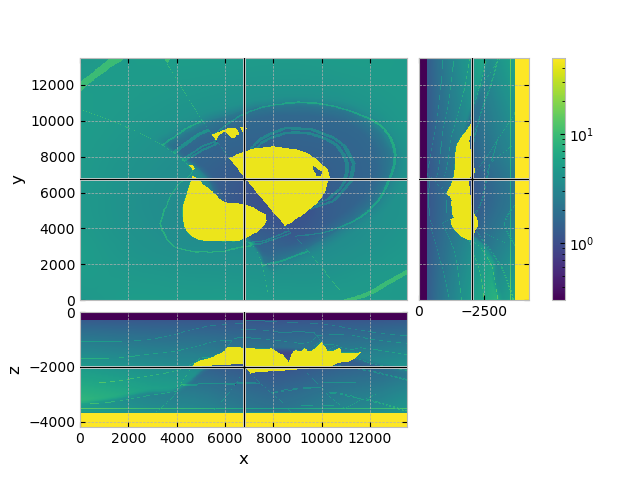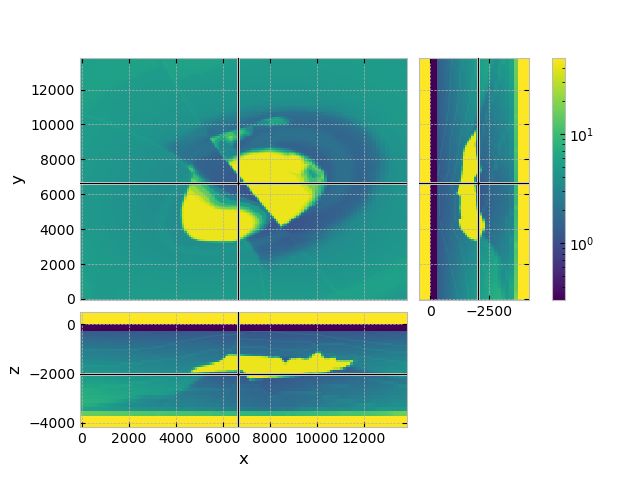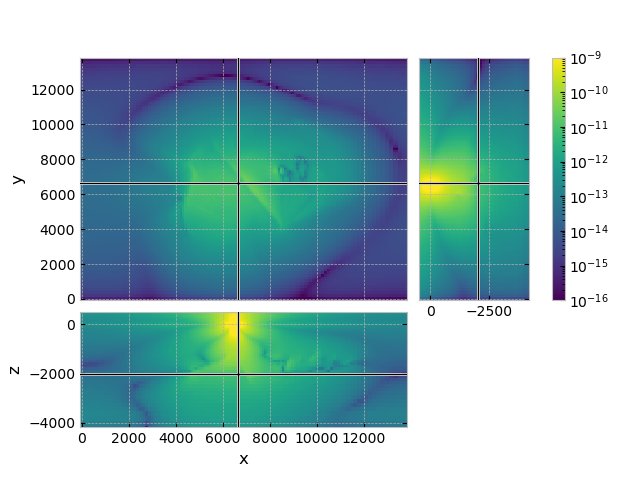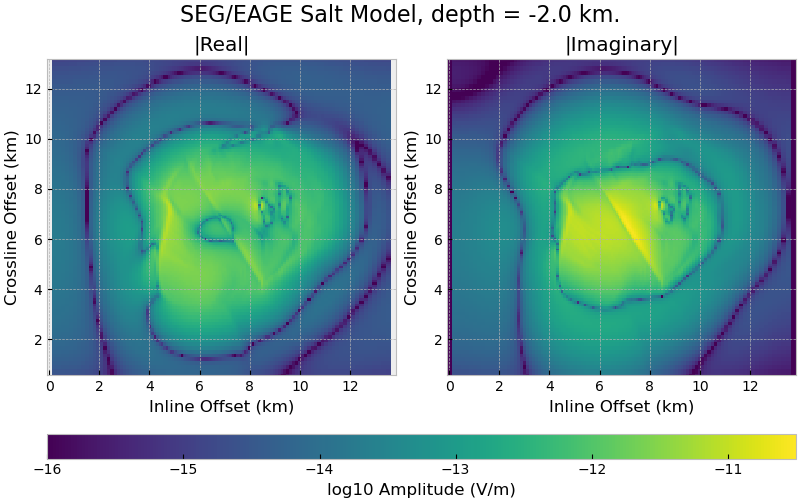# SEG-EAGE 3D Salt Model#

[Muld07] presented electromagnetic responses for a resistivity model which he derived from the seismic velocities of the SEG/EAGE salt model [AmBK97].

Here we reproduce and store this resistivity model, and compute electromagnetic responses for it.

## Velocity-to-resistivity transform#

Quoting here the description of the velocity-to-resistivity transform used by [Muld07]:

«The SEG/EAGE salt model (Aminzadeh et al. 1997), originally designed for seismic simulations, served as a template for a realistic subsurface model. Its dimensions are 13500 by 13480 by 4680 m. The seismic velocities of the model were replaced by resistivity values. The water velocity of 1.5 km/s was replaced by a resistivity of 0.3 Ohm m. Velocities above 4 km/s, indicative of salt, were replaced by 30 Ohm m. Basement, beyond 3660 m depth, was set to 0.002 Ohm m. The resistivity of the sediments was determined by $$(v/1700)^{3.88}$$ Ohm m, with the velocity v in m/s (Meju et al. 2003). For air, the resistivity was set to $$10^8$$ Ohm m.»

Equation 1 of [MeGM03], is given by

(1)#$\log_{10}\rho = m \log_{10}V_P + c \ ,$

where $$\rho$$ is resistivity, $$V_P$$ is P-wave velocity, and for $$m$$ and $$c$$ 3.88 and -11 were used, respectively.

The velocity-to-resistivity transform uses therefore a Faust model ([Faus53]) with some additional constraints for water, salt, and basement.

Note

import os
import pooch
import emg3d
import numpy as np
import matplotlib.pyplot as plt
from matplotlib.colors import LogNorm
plt.style.use('bmh')

# Adjust this path to a folder of your choice.


## Fetch the model#

Retrieve and load the pre-computed resistivity model.

fname = "SEG-EAGE-Salt-Model.h5"
pooch.retrieve(
'https://raw.github.com/emsig/data/2021-05-21/emg3d/models/'+fname,
'6ee10663de588d445332ba7cc1c0dc3d6f9c50d1965f797425cebc64f9c71de6',
fname=fname,
path=data_path,
)
fmodel = emg3d.load(data_path + fname)['model']
fgrid = fmodel.grid

Data loaded from «/home/dtr/3-GitHub/emsig/emg3d-gallery/examples/download/SEG-EAGE-Salt-Model.h5»
[emg3d v1.0.0rc3.dev5+g0cd9e09 (format 1.0) on 2021-05-21T13:15:50.617756].


## QC resistivity model#

# Limit colour-range to [0.3, 50] Ohm.m
# (affects only the basement and air, improves resolution in the sediments).
vmin, vmax = 0.3, 50

fgrid.plot_3d_slicer(
fmodel.property_x,
zslice=-2000,
pcolor_opts={'norm': LogNorm(vmin=vmin, vmax=vmax)}
)## Compute some example CSEM data with it#

### Survey parameters#

# Create a source instance
source = emg3d.TxElectricDipole(
coordinates=[6400, 6600, 6500, 6500, -50, -50],
strength=1/200.  # Normalize for length.
)

# Frequency (Hz)
frequency = 1.0


### Initialize computation mesh#

grid = emg3d.construct_mesh(
frequency=frequency,
properties=[0.3, 1, 2, 15],
center=(6500, 6500, -50),
seasurface=0,
domain=([0, 13500], [0, 13500], None),
vector=(None, None, np.array([-100, -80, -60, -40, -20, 0])),
min_width_limits=([5, 100], [5, 100], [5, 20]),
min_width_pps=5,
stretching=[1.03, 1.05],
lambda_from_center=True,
center_on_edge=True,
)
grid

 MESH EXTENT CELL WIDTH FACTOR dir nC TensorMesh 2,097,152 cells x 128 -71.48 13,830.69 55.13 195.38 1.02 y 128 -71.48 13,830.69 55.13 195.38 1.02 z 128 -4,579.62 12,968.70 20.00 625.54 1.05

### Put the salt model onto the modelling mesh#

# Interpolate full model from full grid to grid
model = fmodel.interpolate_to_grid(grid)

grid.plot_3d_slicer(
model.property_x,
zslice=-2000,
zlim=(-4180, 500),
pcolor_opts={'norm': LogNorm(vmin=vmin, vmax=vmax)}
)### Solve the system#

efield = emg3d.solve_source(
model, source, frequency,
semicoarsening=False,
linerelaxation=False,
verb=1,
)

:: emg3d :: 4.8e-07; 5(10); 0:01:44; CONVERGED

grid.plot_3d_slicer(
efield.fx.ravel('F'),
zslice=-2000,
zlim=(-4180, 500),
view='abs',
v_type='Ex',
pcolor_opts={'norm': LogNorm(vmin=1e-16, vmax=1e-9)}
)# Interpolate for a "more detailed" image
x = grid.cell_centers_x
y = grid.cell_centers_y
rx = np.repeat([x, ], np.size(x), axis=0)
ry = rx.transpose()
rz = -2000
data = efield.get_receiver((rx, ry, rz, 0, 0))

# Colour limits
vmin, vmax = -16, -10.5

# Create a figure
fig, axs = plt.subplots(figsize=(8, 5), nrows=1, ncols=2)
axs = axs.ravel()

titles = [r'|Real|', r'|Imaginary|']

def log10abs(inp):
"""Log10 of absolute values, avoiding zero-division."""
tiny = np.finfo(float).tiny
return np.log10(np.where(abs(inp) < tiny, tiny, abs(inp)))

dat = [log10abs(data.real), log10abs(data.imag)]

for i in range(2):
plt.sca(axs[i])
axs[i].set_title(titles[i])
axs[i].set_xlim(min(x)/1000, max(x)/1000)
axs[i].set_ylim(min(x)/1000, max(x)/1000)
axs[i].axis('equal')
cs = axs[i].pcolormesh(x/1000, x/1000, dat[i], vmin=vmin, vmax=vmax,
plt.xlabel('Inline Offset (km)')
plt.ylabel('Crossline Offset (km)')

# Colorbar
# fig.colorbar(cf0, ax=axs, label=r'$\log_{10}$ Amplitude (V/m)')

# Plot colorbar
cax, kw = plt.matplotlib.colorbar.make_axes(
axs, location='bottom', fraction=.05, pad=0.2, aspect=30)
cb = plt.colorbar(cs, cax=cax, label=r"$\log_{10}$ Amplitude (V/m)", **kw)

# Title
fig.suptitle(f"SEG/EAGE Salt Model, depth = {rz/1e3} km.", y=1, fontsize=16)

plt.show()## QC resistivity model with PyVista#

Note

The following cell is about how to plot the model in 3D using PyVista, for which you have to install pyvista.

The code example was created on 2021-05-21 with pyvista=0.30.1.

import pyvista

dataset = fgrid.to_vtk({'res': np.log10(fmodel.property_x.ravel('F'))})

# Create the rendering scene and add a grid axes
p = pyvista.Plotter(notebook=True)
p.show_grid(location='outer')

dparams = {'rng': np.log10([vmin, vmax]), 'show_edges': False}
# Add spatially referenced data to the scene
xyz = (5000, 6000, -3200)
p.add_mesh(dataset.slice('x', xyz), name='x-slice', **dparams)
p.add_mesh(dataset.slice('y', xyz), name='y-slice', **dparams)
p.add_mesh(dataset.slice('z', xyz), name='z-slice', **dparams)

# Get the salt body
p.add_mesh(dataset.threshold([1.47, 1.48]), name='vol', **dparams)

# Show the scene!
p.camera_position = [
(27000, 37000, 5800), (6600, 6600, -3300), (0, 0, 1)
]
p.show()


## Reproduce the resistivity model#

Note

The last cell as about how to reproduce the resistivity model. For this you have to download the SEG/EAGE salt model, as explained further down.

The code example and the SEG-EAGE-Salt-Model.h5-file used in the gallery were created on 2021-05-21.

To reduce runtime and dependencies of the gallery build we use a pre-computed resistivity model, which was generated with the code provided below.

In order to reproduce it yourself you have to download the data from the SEG-website or via this direct link. The zip-file is 513.1 MB big. Unzip the archive, and place the file Salt_Model_3D/3-D_Salt_Model/VEL_GRIDS/SALTF.ZIP (20.0 MB) in the same directory as the notebook.

The following cell loads takes this SALTF.ZIP, carries out the velocity-to-resistivity transform, and stores the resistivity model for later use.

import emg3d
import zipfile
import numpy as np

# Dimension of seismic velocities
nx, ny, nz = 676, 676, 210

# Create a discretize-mesh of the correct dimension
# (nz: +1, for air)
fgrid = emg3d.TensorMesh(
[np.ones(nx)*20., np.ones(ny)*20., np.ones(nz+1)*20.],
origin=(0, 0, -210*20))
res = np.zeros(fgrid.shape_cells, order='F')

zipfile.ZipFile('SALTF.ZIP', 'r').extract('Saltf@@')
with open('Saltf@@', 'r') as file:
v = np.fromfile(file, dtype=np.dtype('float32').newbyteorder('>'))
res[:, :, 1:] = v.reshape((nx, ny, nz), order='F')

# Velocity to resistivity transform for whole cube
res = (res/1700)**3.88  # Sediment resistivity = 1

# Overwrite basement resistivity from 3660 m onwards
res[:, :, np.arange(fgrid.shape_cells)*20 > 3680] = 500.

# Set sea-water to 0.3
res[:, :, :16][res[:, :, :16] <= 1500] = 0.3
# Ensure at least top layer is water
res[:, :, 1] = 0.3

# Fix salt resistivity
res[res == 4482] = 30.

# Set air resistivity
res[:, :, 0] = 1e8

# THE SEG/EAGE salt-model uses positive z downwards; discretize positive
# upwards. Hence for res, we use np.flip(res, 2) to flip the z-direction
res = np.flip(res, 2)

# Create the resistivity model
model = emg3d.Model(fgrid, property_x=res)

# Store the resistivity model
emg3d.save("SEG-EAGE-Salt-Model.h5", model=model)

emg3d.Report()

 Wed Aug 31 21:51:30 2022 CEST OS Linux CPU(s) 4 Machine x86_64 Architecture 64bit RAM 15.5 GiB Environment Python File system ext4 Python 3.9.12 | packaged by conda-forge | (main, Mar 24 2022, 23:22:55) [GCC 10.3.0] numpy 1.22.4 scipy 1.9.0 numba 0.55.2 emg3d 1.8.0 empymod 2.2.0 xarray 2022.6.0 discretize 0.8.2 h5py 3.7.0 matplotlib 3.4.3 tqdm 4.64.0 IPython 8.4.0 Intel(R) oneAPI Math Kernel Library Version 2022.0-Product Build 20211112 for Intel(R) 64 architecture applications

Total running time of the script: ( 1 minutes 55.776 seconds)

Estimated memory usage: 2752 MB

Gallery generated by Sphinx-Gallery